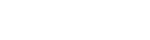# Rebalance Probabilities

Upvote
8
Downvote
Save
Mark Completed
Have you seen this question before?

Suppose we are training a binary classification algorithm.

99.8% of individuals in our sample have a value of 0 and 0.2% have a value of 1. We downsample and we randomly sample say 1% of the individuals which have a value of 0 but keep all other individuals with a value of 1.

Now we re-train the model on the smaller sample and build a binary classifier that predicts the probability of an individual with a value of 1.

How would we then adjust our output probabilities to use this model on the total population?

Next question: Random Forest Explanation
.....